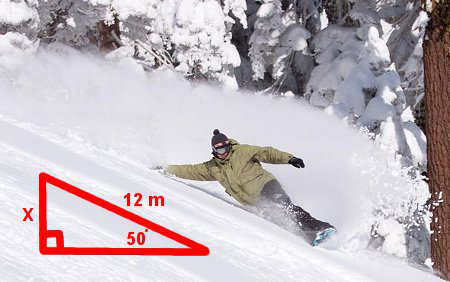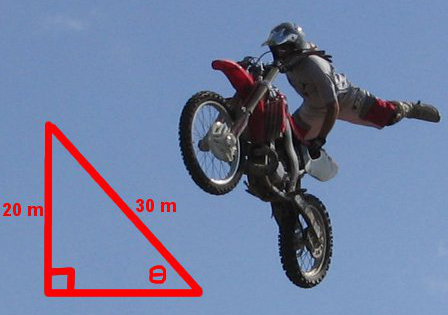# Trigonometry Sine Rule

There are 3 trigonometry rules which are:

• Sin θ = O/H
• Cos θ = A/H
• Tan θ = O/A (when there is no hypotenuse)

## Example One - Sine Rule to Find the Opposite SideA mountain slope has a sloped length of 12 metres and an angle of 50° that the slope makes with level ground. What is the vertical height of the slope?

sin θ = O/H

(Always draw a diagram and write the rule. Then substitute the numbers and letters specific to this question. See the next line of working.)

sin 50 = x/12
(If x is on the top of the fraction, multiply both sides of the equation by the number on the bottom which is 12.)

sin 50 × 12 = x
(Now type sin 50 × 12 on your calculator. You may need to close the bracket after the 50.)

x = 9.19

The vertical height of the track is 9.19 metres.

## Question - Finding the Opposite Side

Another mountain has a sloped length of 32 metres and an angle of 40° that the slope makes with level ground. What is the vertical height of the slope? (Draw a diagram.)

20.57 metres

## Example Two - Sine Rule to Find the Hypotenuse

A cable car at a ski resort carries skiers up to the top of the mountain. It is supported by a steel cable. The vertical height of the mountain is 3000 metres and the angle that the cable makes with the ground is 40°. What is the sloped length of the cable?sin θ = O/H
(Draw a diagram and write the rule. Then substitute the numbers and letters specific to this question. See the next line of working.)

sin 40 = 3000/x
(If x is on the bottom of the fraction, multiply both side by x and divide both sides by sin 40. In effect, you will swap the sin and the side. See the next line of working.)

x = 3000 / sin 40
(Type 3000 / sin 40 on your calculator. You may need to close the bracket after the 40.)

x = 4667.17 metres

The sloped length of the cable must be 4667.17 metres.

## Question - Finding the Hypotenuse

Another snowy slope has a vertical height of 1800 metres and the cable angle is 30°. What is the sloped length of the cable? (Draw a diagram.)

3600 metres

## Example Three - Sine Rule to Find an AngleFor a motorbike stunt, stuntman Wild Ram Bo must ride at high speed up a ramp with a vertical height of 20 metres and a sloped length of 30 metres. What is the angle with the ground?

sin θ = O/H
(Draw a diagram and write the rule. Then substitute the numbers and letters specific to this question. See the next line of working.)

sin θ = 20/30

θ = sin-1 (20/30)
(Type Shift Sin (20/30) on your calculator. Use brackets for the fraction part.)

θ = 41.81°

The angle of the stunt ramp is 41.81°.

## Question - Finding an Angle

As for the bad news, the stunt didn't work. The good news is that the Ram is recovering in hospital. The substitute rider, Wilder Spin, constructed a new ramp with a vertical height of 25 metres and the same sloped length of 30 metres. What is the angle with the ground? (Draw a diagram.)